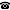# 2019 seminar talk: Chromatic numbers of finite subgraphs

Talk held by Christopher Lambie-Hanson (Virginia Commonwealth University, Richmond, USA) at the KGRC seminar on 2019-03-14.

### Abstract

By the De Bruijn-Erdős Compactness Theorem, if a graph $G$ has infinite chromatic number, then it has finite subgraphs of arbitrarily large finite chromatic number. We can therefore define an increasing function $f_G:\omega \rightarrow \omega$ by letting $f_G(n)$ be the least number of vertices in a subgraph of $G$ with chromatic number $n$. We will show in ZFC that, for every function $f:\omega \rightarrow \omega$, there is a graph $G$ with chromatic number $\aleph_1$ such that $f_G$ grows faster than $f$. This answers a question of Erdős, Hajnal, and Szemeredi. Time permitting, we will discuss connections between our proof and various diamond and club-guessing principles.

A video recording of this talk is available on YouTube.

Kurt Gödel Research Center for Mathematical Logic. Währinger Straße 25, 1090 Wien, Austria.+43-1-4277-50501. Last updated: 2010-12-16, 04:37.